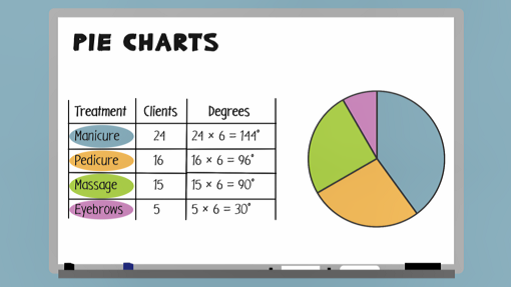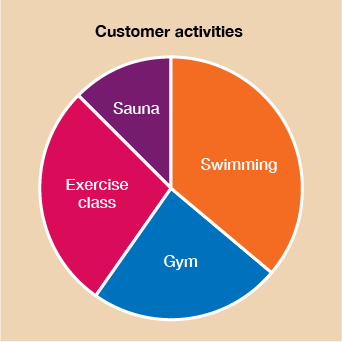Everyday maths 2

Start this free course now. Just create an account and sign in. Enrol and complete the course for a free statement of participation or digital badge if available.

Free course

# 4.1 Drawing pie charts

The best way to understand the steps involved in drawing a pie chart is to watch the worked example in the video below.Skip transcript

#### Transcript

When you draw a pie chart, you're splitting a circle into a number of different sections of different sizes, depending on the data you're given. A full circle has a total of 360 degrees. A quarter of a circle is 90 degrees, and a half circle is 180 degrees.

Let's create a pie chart to show you the steps involved in drawing one. This table shows some information about the number of clients at a beauty salon and what treatments they had. If you want to draw a pie chart, you need to know how many degrees of the circle to allocate for each treatment.

To do this, you need to divide 360 degrees by the number of clients. This is because you want to share the full circle, 360 degrees, equally between each of the clients. Each person is represented by a certain number of degrees.

In this example, the total number of clients equals 24 + 16 + 15 + 5, which equals 60. 360 divided by 60 equals 6, so each client gets six degrees of the circle.

Now that you know how many degrees each treatment type is worth, you can draw the pie chart. First, you need to draw a circle. Next, draw a line from the centre of the circle to the top of the circle. This will be the line that you start drawing your slices from. Now, use a protractor to measure the correct angles and draw the pie chart.

It should look something like this. Now, have a go at drawing a pie chart for yourself.

End transcript

Interactive feature not available in single page view (see it in standard view).

Now have a go at drawing a pie chart for yourself.

## Activity 5: Drawing a pie chart

A leisure centre wants to compare which activities customers choose to do when they visit the centre. The information is shown in the table below. Draw an accurate pie chart to show this information.

### Table 7

ActivityNumber of customers
Swimming26
Gym17
Exercise class 20
Sauna9

Firstly, work out the total number of customers: 26 + 17 + 20 + 9 = 72.

Now work out the number of degrees that represents customer: 360˚ ÷ 72 = 5˚ per customer.

#### Table 8

ActivityNumber of customersNumber of degrees
Swimming2626 × 5 = 130˚
Gym1717 × 5 = 85˚
Exercise class 2020 × 5 = 100˚
Sauna99 × 5 = 45˚

Now use this information to draw your pie chart. It should look something like this:Figure 10 Pie chart for customer leisure centre activities

Now that you can accurately draw a pie chart, it’s time to look at how to interpret them. You won’t always be given the actual data, you may just be given the total number represented by the chart or a section of the chart and the angles on the pie chart itself. It’s useful to know how to use your maths skills to work out the actual figures.

Here’s a reminder of the degrees of a circle which will be useful when you come to read from pie charts.Figure 11 Degrees of a circle
FSM_2